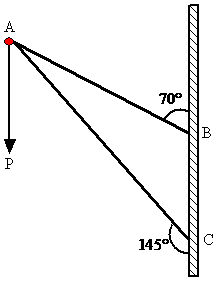Visit the website 123iitjee.manishverma.site for latest posts, courses, admission & more.

###Consider a frame that is made up of two thin massless rods AB and AC as shown in the figure. A vertical force $\vec P$  of magnitude 100N is applied at point A of the frame. Suppose the force $\vec P$  is resolved parallel to the arms AB and AC of the frame. The magnitude of the resolved component along the arm AC is xN. The value of x, to the nearest integer, is------------.

[Given: $\sin 35^\circ = 0.573,{\rm{ }}\cos 35^\circ = 0.819,{\rm{ }}\sin 110^\circ = 0.939,{\rm{ }}\cos 110^\circ = - 0.342$]

Solution

Let $F_1$  and $F_2$  be the components of force P along AC and BA respectively.

So, ${F_1}\sin 35^\circ = {F_2}\sin 70^\circ$  (as P does not have any horizontal component)

$\Rightarrow {F_2} = {F_1}\frac{{\sin 35^\circ }}{{\sin 70^\circ }}$

Also, $P = {F_1}\cos 35^\circ - {F_2}\cos 70^\circ$  (vertical direction)

$\Rightarrow P = {F_1}\cos 35^\circ - {F_1}\frac{{\sin 35^\circ }}{{\sin 70^\circ }}\cos 70^\circ = {F_1}\frac{{\sin (70^\circ - 35^\circ )}}{{\sin 70^\circ }} = {F_1}\frac{{\sin 35^\circ }}{{\sin 70^\circ }}$

$\Rightarrow {F_1} = P\frac{{\sin 70^\circ }}{{\sin 35^\circ }} = 2P\cos 35^\circ = 2 \times 100 \times 0.819 = 163.8 \approx 164N$

### Sum of the coefficients in the expansion of $(x+y)^n$ ....

If the sum of the coefficients in the expansion of $(x+y)^n$ is 4096, then the greatest coefficient in the expansion is _ _ _ _ . Solution $C_0 + C_1 + C_2 + C_3 + ......................... + C_n =4096$ $\therefore 2^n = 4096 =2^{12}$ $\Rightarrow n = 12$ Greatest coefficient = ${}^{12}{C_6} = 924$